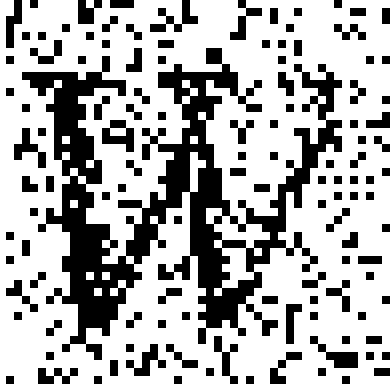# Perfect animations of conditioned Ising models

## Introduction

Here is a simple example of how perfect (or exact) simulation for the Ising model may be used in statistical image analysis.
Suppose we are given a binary image which has been corrupted by noise:We model this as follows: the "true" colour of a given pixel depends only on its four nearest neighbours, and the observed pixel colour depends directly only on the "true" pixel colour. We omit the exact specification of the model, but it leads to the following: if we code black by +1 and white by -1 then the conditional or posterior probability of a "true" pixel colour having code +1 (given its neighbours and the observed image) is proportional to

```exp(+ (beta*(sum of neighbouring "true" pixel codes)/2 + phi*(observed pixel code)))
```

while the conditional probability of the colour having code -1 is proportional to

```exp(- (beta*(sum of neighbouring "true" pixel codes)/2 + phi*(observed pixel code))).
```

For the purposes of illustration, we take

```beta=2, phi=2.
```

## MCMC and perfect simulation

We can sample approximately from the posterior distribution by running a specific "heat bath" Markov chain till it is in approximate equilibrium; scanning through the image pixel by pixel and replacing each pixel by a draw from the conditional distribution as specified above. In 1996 Propp and Wilson noted that this approximate simulation algorithm can be converted into an exact algorithm as follows: consider the simulation run as started at time -T with unspecified initial data and run till time 0. Here is an informal summary of their idea: continue increasing T (thus beginning the simulation earlier and earlier, and re-using randomness at specific times) till the output at time 0 no longer depends on the initial state (which is to say, there is coalescence); the output at time 0 will be then an exact draw from the posterior distribution. This is the idea of perfect (or exact) simulation.
We can ascertain when the output no longer depends on the input at time -T by the simple expedient of comparing the results when spins at time -T are either all +1 or all -1; monotonicity of the heat bath algorithm allows us to deduce that all results at time 0 will agree if these two do.
Here is a GIF animation (2.1 MB) illustrating this Coupling from the Past algorithm (CFTP): click on the image to open the animation page or download it by right-clicking on this linkand run it locally (better if your internet connection is slow!). We follow the "binary search" approach of doubling T each time we repeat the cycle of simulation from time -T to time 0.

## CFTP viewed as separate cycles

There are several different ways to view the results of a CFTP algorithm, which after all produces a somewhat involved data structure, with time extending backwards rather than forwards! Here is a table of links to the four separate component cycles of the above CFTP run which occur prior to coalescence, presented as animated GIFs up to the point where coalescence is obtained at time 0. Click on the images to start the animations (if your internet connection is slow it may be better to right-click on the image to download to your machine to run locally).

 Starting at time -16 (0.5 MB) Starting at time -32 (0.5 MB) Starting at time -64 (0.5 MB) Starting at time -128 (0.6 MB) and achieving coalescence at time 0

Note that the "binary search" approach of doubling T could be finessed here - though the above output can be misleading. The last few steps of CFTP can be necessarily expensive, as indicated in the next and final section.

W.S. Kendall, 21 January 2004